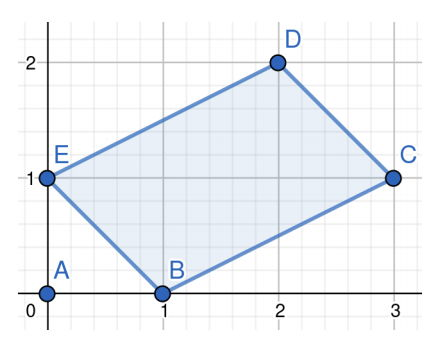시간 제한 메모리 제한 제출 정답 맞은 사람 정답 비율
6 초 1024 MB 14 5 5 55.556%

## 문제

Given some points on a 2D Euclidean plane, please calculate the maximum area of quadrilaterals with vertices in given points. For example, points A(0, 0), B(1, 0), C(3, 1), D(1, 2), E(0, 1) are given. These points form 5 simple quadrilaterals ABCD, ABCE, ABDE, ACDE, BCDE with areas 3, 2, 1.5, 3, 3 respectively and 10 complex quadrilaterals ABDC, ABEC, ABED, ACED, BCED, ACBD, ACBE, ADBE, ADCE, BDCE with smaller areas. So the maximum area is 3.Figure 4: BCDE has the maximum area 3 among all quadrilaterals.

Please notice that duplicated points may appear in the given points. All the degenerate cases are also taken as quadrilaterals such as quadrilateral ABCD with A(0, 0), B(0, 0), C(0, 0), D(0, 0).

## 입력

The first line is an integer T indicating the number of test cases. The first line of each test case contains an integer N followed by N lines. Each line of the following N lines contains two integers X and Y representing a point (X, Y ).

## 출력

For each test case, please output the maximum area among all quadrilaterals with vertices in given points.

## 제한

• 1 ≤ T ≤ 3
• 4 ≤ N ≤ 4096
• 0 ≤ X ≤ 109
• 0 ≤ Y ≤ 109
• You may not output numbers with scientific notaions. I.e., outputting 3E8 for 300000000 is not allowed.
• The area must be outputted without any redundant characters. I.e., outputting 3.0 for 3 is incorrect.

## 예제 입력 1

3
5
0 0
1 0
3 1
1 2
0 1
4
0 0
4 0
0 4
1 1
4
0 0
1 1
2 2
1 1


## 예제 출력 1

3
6
0


## 예제 입력 2

1
4
0 0
1 0
0 1
3 2


## 예제 출력 2

2.5